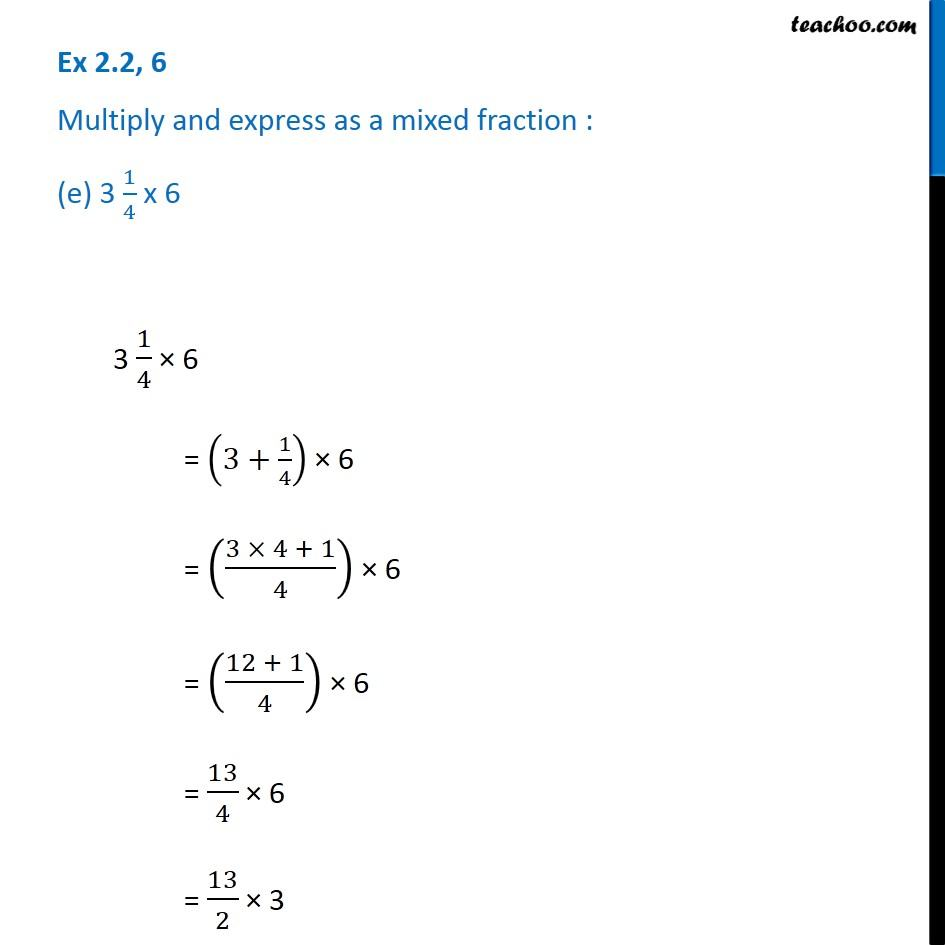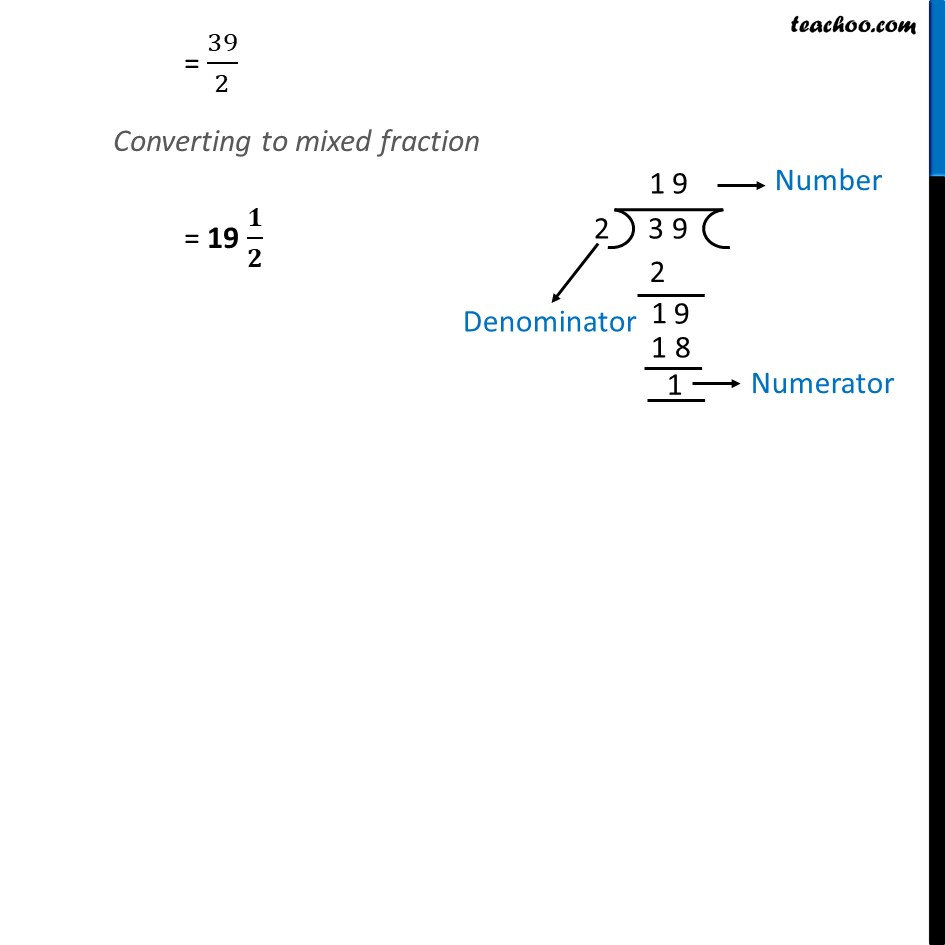1. Chapter 2 Class 7 Fractions and Decimals
2. Serial order wise
3. Ex 2.2

Transcript

Ex 2.2, 6 Multiply and express as a mixed fraction : (e) 3 1/4 x 6 3 1/4 × 6 = (3+1/4) × 6 = ((3 × 4 + 1)/4) × 6 = ((12 + 1)/4) × 6 = 13/4 × 6 = 13/2 × 3 = 39/2 Converting to mixed fraction = 19 𝟏/𝟐